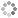# PROWAREtecharticles » current » javascript » three-js » orbit-a-body

## ThreeJS: How to Orbit a Body

How to make a 3D/mesh object (the satellite) orbit another object (the body) using THREE.js; how to make a celestial/solar system.

This simple code snippet is all that is needed to make one object (orbiter) orbit another object (body):

``````
// NOTE: speed less than 0 will cause the direction of spin to change; alternatively, multiply the output of cosine by -1
var orbitBody = function (body, orbiter, speed, distanceFromBody) {
var time = Date.now();
orbiter.position.x = Math.cos(time * speed / 1000) * distanceFromBody + body.position.x;
orbiter.position.z = Math.sin(time * speed / 1000) * distanceFromBody + body.position.z;
}
``````

Here is a full working example:

``````
<!DOCTYPE html>
<html lang="en">
<meta charset="UTF-8">
<meta http-equiv="X-UA-Compatible" content="IE=edge">
<meta name="viewport" content="width=device-width, initial-scale=1.0">
<title>Orbiting</title>
<style>
body {
background-color: white;
}
canvas {
display: block;
width: 300px;
height: 300px;
}
</style>
<body>
<canvas></canvas>
<script src="/js/three.min.js"></script>
<script type="text/javascript">

(function () {

var canvas = document.getElementsByTagName("canvas");

// NOTE: create the scene to place objects in
var scene = new THREE.Scene();
scene.background = new THREE.Color(0x6699CC);
scene.matrixWorldAutoUpdate = true;

// NOTE: the width and height of the canvas
var size = {
width: canvas.offsetWidth,
height: canvas.offsetHeight
};

var cameraNear = 1, cameraFar = 500;
var camera = new THREE.PerspectiveCamera(40, size.width / size.height, cameraNear, cameraFar);

// NOTE: position the camera in space a bit
camera.position.z = 18;

var renderer = new THREE.WebGLRenderer({
canvas: canvas,
antialias: true
});
renderer.setPixelRatio(window.devicePixelRatio);
renderer.setSize(size.width, size.height);
renderer.render(scene, camera);

var light = new THREE.DirectionalLight(0xFFFFFF, 1);
light.position.set(2, 2, 2);

var sphereGeometry1 = new THREE.SphereGeometry(sphereRadius1, 16, 16);

var sphereGeometry2 = new THREE.SphereGeometry(sphereRadius2, 16, 16);

var sphereGeometry3 = new THREE.SphereGeometry(sphereRadius3, 16, 16);

var material = new THREE.MeshLambertMaterial({ color: 0xCC6633 })

var group = new THREE.Group();

var sphere1 = new THREE.Mesh(sphereGeometry1, material);

var sphere2 = new THREE.Mesh(sphereGeometry2, material);

var sphere3 = new THREE.Mesh(sphereGeometry3, material);

// NOTE: speed less than 0 will cause the direction of spin to change; alternatively, multiply the output of cosine by -1
var orbitBody = function (body, orbiter, speed, distanceFromBody) {
var time = Date.now();
orbiter.position.x = Math.cos(time * speed / 1000) * distanceFromBody + body.position.x;
orbiter.position.z = Math.sin(time * speed / 1000) * distanceFromBody + body.position.z;
}

var animate = function () {

orbitBody(sphere1, sphere2, 1, 5); // NOTE: better to move sphere2 first
orbitBody(sphere2, sphere3, -3, 1);

renderer.render(scene, camera);
requestAnimationFrame(animate);
};
animate();

})();

</script>
</body>
</html>
``````This site uses cookies. Cookies are simple text files stored on the user's computer. They are used for adding features and security to this site. Read the privacy policy.